# HANDWRITING RECOGNITION USING CNN – AI PROJECTS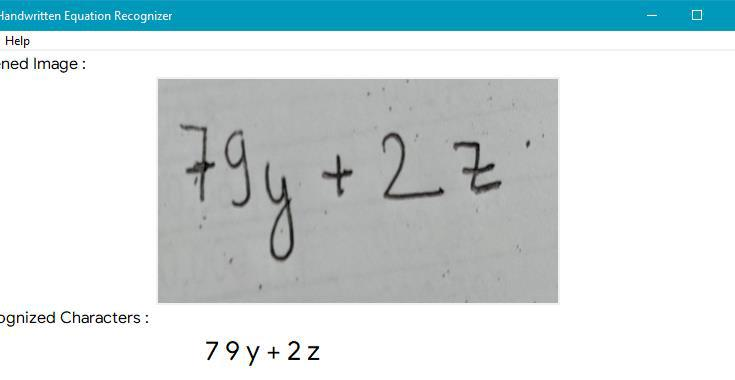Machine Learning is an application of artificial intelligence (AI) that provides systems the ability to automatically learn and improve from experience without being explicitly programmed. It can also be defined as the scientific study of algorithms and statistical models that computer systems use to effectively perform a specific task without using explicit human intervention, relying on patterns and inference instead. Similarly, a mathematical equation is a statement that defines the equality of two expression which can be used to define almost all the remaining mathematical theorems and science theories.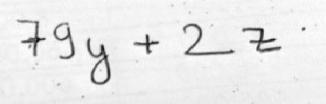Fig: Mathematical Equation

Neural Networks are simply an artificial model of the human brain which are generally composed of perceptron which are further composed of structures known as nodes and weights. These nodes can activate or deactivate with inputs and further activate more nodes further levels down the neural path. This is the basic concepts by which neural network works. In deep learning, a convolutional neural network (CNN or ConvNet) is a class of deep neural network, most commonly applied to visual imagery. CNNs are regularized versions of multilayer perceptrons. Multilayer perceptrons are usually referred to fully connecter networks, that is each neuron in one layer is connected to all neurons in the next layer.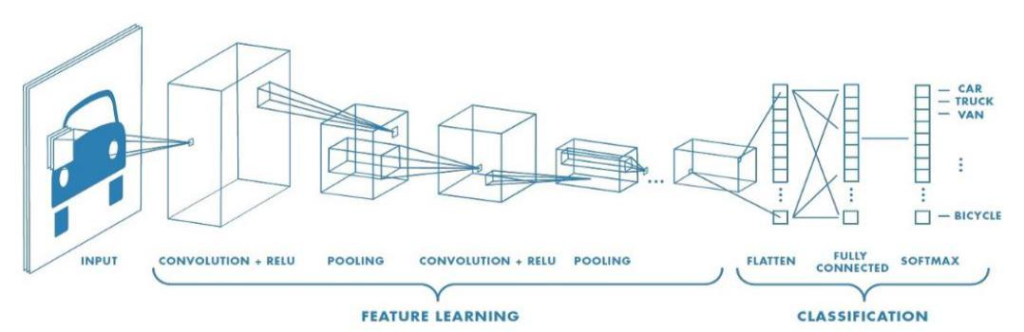Example of Convolution Neural Network

Handwritten Equation Recognizer is a software program written to ease the process of recognizing the characters that comprises in any given mathematical equations. It involves just the use of manual photo capture of the mathematical equation and feeding the photo to the software to obtain the characters the mathematical equation is comprised of for further processing and calculation. For a normal human being, pattern recognition is the most basic of the human learning concepts which comes as a second nature to most of us. The same is not true for computers and machines. To identify patterns and be able to compute on the information that is obtained through the identification of the pattern is a great accomplishment of a computer. This poses a great challenge in making a computer to be able to understand and parse mathematical expressions which in turn are typical example of pattern recognition. Being able to design a neural network that can successfully ‘teach’ a computer to recognize mathematical patterns so that it can finally carry out further computation on the expression automatically or with least manual input from humans will most certainly bring about a great ease in mathematical equations calculations.

The software created on the end of this project work aims to do just the things mentioned above. It trains a computer model to recognize mathematical patterns and is able to return to the user a list of characters that are involved in making the equation.

#### Problem Statement

The situational problems that exists with current similar implementation of Handwritten Equation Recognizer have numerous problems like not being based on pure machine learning which won’t be able to take advantage of the huge processing power of the machines that exist in today’s world, and not having enough data to accurately predict the characters that are extracted from the image being fed into the software. Additionally, using conventional way of computing equations are also not productive due to requiring huge amount of manual input from the user.

Further problems encountered in manual input is that due to complex nature of certain problems there can occur user error during manual input of data inside a calculator. This causes discrepancies and errors in the obtained data and can cause devastating effect on some fields. Handwriting Equation Recognizer solves this problem by requiring very less.

#### Scope

After this project work will have completed, the software can be used to convert mathematical equations into computer readable form which in turn can be written directly down for further calculations or simply data entry in any software solutions. It also presents the characters in an easy to understand pattern that can be further improved to carry out calculation and further train to be able to recognize equations in multiple language after feeding the data in the model. This project can also be implemented in other complex projects such as online calculators which will be able to just take the image of the equation and compute the solution for this equation without additional human input and intelligence. This has number of advantages which mainly lead to saving of time during such equation solution which will certainly be helpful for many fields which currently have a schedule to go with the time loss in solving and parsing such equations. Using the high computation power of current computers and the result of this project even complex solutions of equations can be solved in matter of seconds.

Hence after completion of this project, it can be released which in turn can be used by many people for purposes like parsing, extraction, recognition and calculation of characters comprising of the equation and also for further use in personal or advanced projects.

System Requirements

The main system requirements for the Handwritten Equation Recognizer using

Convolution Neural Network are mentioned below:

1. A CUDA (Compute Unified Device Architecture) Application Programming Interface which will need to provide GPU (Graphical Processing Unit) accelerated neural network for high performance and high-speed model.

1. An Anaconda3 installation with Python interpreter for actual code interpreting and important machine learning and deep learning libraries.

1. A labeled dataset consisting of the numbers and characters used in mathematical equations to train the model for accuracy.

1. Visual Studio Code, which is the main code editor for writing the program.

1. Various Additional Libraries that are used for programming. For this project we used Numpy, Pytorch, Scikit-Learn, Open-CV2, tKinter, and Matplotlib.

Additionally, the above requirements are for training the model, for the implementation phase the only requirements are a computer having a microprocessor capable of at least running on the clock speed of 1.2 GHz.

Algorithms

Handwritten Equation Recognizer’s complete working was divided into two parts- training phase and implementation phase. Both parts include methods of image preprocessing but only the implementation phase consists of segmentation and re-assembly. Hence the following are the algorithms used for various mentioned processes.

Training Phase

Training Phase consists of Data Preprocessing, Model Training and Loss Calculation whose algorithms are mentioned below:

Data Preprocessing

The Algorithm for data preprocessing is given below:

1. Import necessary Libraries and API (For our project, numpy, os, cv2, random, and matplotlib was imported)

1. Create a Label for each folder where the data is present. For our project there are 30 folders each with 24 unique characters used in our recognition system. Each folder consists of only the characters data which is denoted by the folder’s name. Hence for ‘-‘ image, the folder it is contained in is named ‘-‘. Store the labels in a variable with an identifier number (index).

1. Merge the image with the identifier using numpy and shuffle the data.

1. Now split the dataset into “HerX.npy” with all the image data and “HerY.npy” with all the labels for training.

1. Finally, make a separate numpy file “Labels_her.npy” with link to each identifier and image label. Example: For “1” our identifier is 5 so we link “1” with 5 so whenever 5 is predicted from the model, it is actually “1”. We do this for all 55 characters.

Model Training and Loss Calculation

The Algorithm for Model Training and Loss Calculation is given below:

1. Import Necessary Libraries and API (For our project, Pytorch, and its neural network libraries, sklearn data splitter, dataloader for batch processing, and CUDA library).

1. Define the Model using Pytorch class and initiate the model into “net”.

1. Load the two .npy files with images and labels and normalize the image data by shrinking its data between 0 and 1.

1. Split the loaded data into training data and testing data. (33% of total data is testing data, the rest is training data)

1. Create Tensor variables for each of the four variables as obtained from 4 for Pytorch CNN input.

1. Split the data into batches of 300 (our project) without shuffling for faster and efficient training.

1. Define the Learning rate and total epochs for training. (For our project Learning rate = 0.001 and total Epochs are = 1000

1. Define the optimizer and loss calculation function for our backpropagation for training. (We used Cross Entropy Loss and Adam Optimizer).

1. Compute the total training steps required and initialize correct and total data parsed to 0.

1. Start the training loop for total epochs.

1. For every I, (images, labels) in count and combine train_X_data_tensor and train_Y_data_tensor.

1. Feed every image to model “net” and store the obtained outputs to “outputs”.

1. Compute the loss from variable defined in 8 and store it in “loss”.

1. Equalize all gradients in the model to zero.

1. Carry out Backward Propagation and compute sensitivity.

1. Update the weights of the Model “net”.

1. Check if predicted data is equal to the actual label of the data and if it is add 1 to correct.

2. Increase total by 1.

3. Display all loss and accuracy after every 100 batches of images have been processed.

4. Go to 11 until all the images are processed else go to 21.

5. Go to 10 until all epochs are completed else go to 22.

6. Initialize the model “net” to evaluation mode.

7. Erase all the gradients from the model.

8. Redefine correct and total and equate both to 0.

9. For every I, (images, labels) in count and combine test_X_data_tensor and test_Y_data_tensor.

10. Feed every image to model “net” and store the obtained outputs to “outputs”.

11. Check if predicted data is equal to the actual label of the data and if it is, add 1 to correct.

12. Increase total by 1

13. Go to 25 until all the images are processed else go to 30.

14. Display the test accuracy of the model.

15. Save the model to “her_model”.This way the training was performed.

Implementation Phase

The Implementation Phase consists of Image Preprocessing, Segmentation, Prediction and Re-Assembly process which are mentioned below:

1. Import Necessary Libraries and APIs. (For this phase, cv2, numpy, skimage.filters, pytorch and os libraries were used.)
2. Define the Model using Pytorch class.

3. Initiate the model into “net”.

4. Load the saved model.

5. Get the input image from the user.

6. Resize the Image into appropriate resolution (400 x 224) and convert it into grayscale.

7. Remove Noise from the image and increase contrast of only the handwritten characters.

8. Remove the background using kernel.

9. Find Contours in the image using mean and standard deviation.

10. Using the data from 9 compute the positions of the characters and the number of characters.

11. Define the rectangle to enclose the different characters in the preprocessed image.

12. Split the different rectangles with separated characters

13. Increase the padding of the individual separate images.

14. Feed the image into the model “net” to compute the output.

15. Sort the outputs according to the position of the rectangle.

16. Display these output values to the user.

17.

Model Description

The Convolution Neural Network model of Handwritten Equation Recognizer consists of 7 total layers. Each of the seven layers are detailed below:

First Layer is the Input Layer which consists of nodes of 45×45 inputs. This is the actual number of pixels in the image that is being input into the model. Each of this pixel have individual values of floating point constants ranging from 0 to 1. The next layer is the first of the Convolution layer with 32 layers that are split to distinguish features from the input image. The layer consists of 5×5 Filter layer with Leaky ReLU as activation function with padding 3 and a 2×2 Max Pooling layer with stride 2. The output from this layer is fed into the second layer.

The second layer is another of the Convolution layers with 64 layers for feature extraction with similar 5×5 filter with padding 3 and 3×3 max pooling layer with Leaky ReLU as activation function and stride = 2. This output is then fed into another 3rd convolution layer.

The third convolution layer consists of 128 layers splits with 5×5 convolution filter with Leaky ReLU with padding = 3 and 3×3 Max Pooling layer with stride = 2. Finally, the output of this layer is sent to the drop-out function.

Drop-out function is a function used using Convolution Neural Network training to avoid the overfitting situation that arises when there is large number of nodes for less data which when not dropped-out can make the model remember each and every data reducing the overall effectiveness of the prediction accuracy of the model. Before the drop-out function, the output is also reshaped or rather Flattened for easy processing of data for the next layer.

The output of the drop-out function is then fed into the first of the fully connected layer but the fourth layer with 128 x 7 = 896 nodes which are then connected to the fifth layer and sixth layer with 500 and 250 nodes respectively.

Finally, the sixth layer is connected to the final seventh and output layer with 24 outputs which predicts the character in the input image. This process is same for both training and implementation phase. The convolution neural network model used in Handwritten Equation Recognizer is displayed more effectively in the diagram below: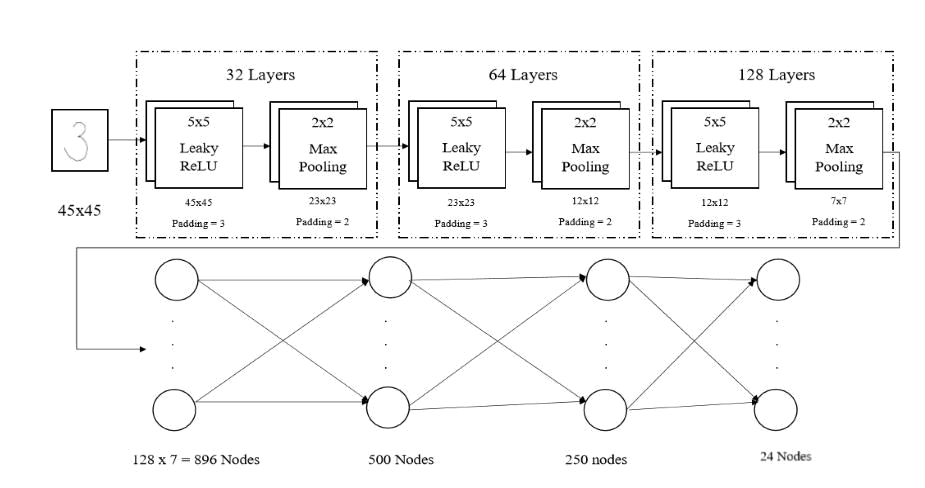RESULT AND ANALYSIS

After the completion of the project we obtained the following results:

1. The accuracy of Training was found to be 95.88%.

1. The accuracy of Testing was found to be 97.14%.

These accuracies denote very good performance of our created model. We additionally created the Graphical User Interface which the user can interact with to operate our software project. Some screenshots of Handwritten Equation Recognizer are given below: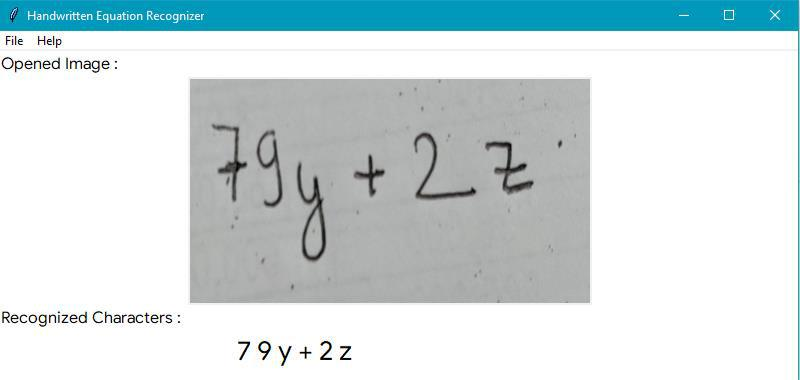REFERENCES

Books

• Russel, Stuart and Peter Norvig. Artificial Intelligence: A Modern Approach Second Edition. Dorling Kindersley. India. 2011.

Research Papers

• Gupta, Prachi, Neelam Pal, Lavanya Agrawal. Recognition of Handwritten Mathematical Equations. IMS Engineering College. India. 2017.

Accessed on: 16th May, 2019 4:40 pm

• Mohapatra, Hitesh. Handwritten Character Recognition (HCR) using Neural Network. Veer Surendra Sai University of Technology. India. 2009.

Accessed on: 24th May, 2019 8:00 am

• Lu, Catherine, Karanveer Mohan, Recognition of Online Handwritten Mathematical Expression Using Convolution Neural Networks. Stanford University.

Accessed on: 24th May, 2019 8:00 am

Websites

• http://www.willforfang.com/computer-vision/2016/4/9/artificial-intelligence-for-handwritten-mathematical-expression-evaluation

Accessed on: 24th May, 2019 7:55 am

• https://towardsdatascience.com/ Accessed on: 20th May, 2019 9:10 am

• https://en.wikipedia.org/wiki/Google_Translate Accessed on: 28th May, 2019 8:50 pm

• https://mathpix.com/about

Accessed on: 28th May, 2019 9:00 pm

• https://en.wikipedia.org/wiki/Optical_character_recognition Accessed on: 28th May, 2019 9:20 pm

## DOWNLOAD APP NOW !!

Page Title#### About Diwas Pandey

Highly motivated, strong drive with excellent interpersonal, communication, and team-building skills. Motivated to learn, grow and excel in Data Science, Artificial Intelligence, SEO & Digital Marketing

View all posts by Diwas Pandey →

### 6 Comments on “HANDWRITING RECOGNITION USING CNN – AI PROJECTS”

1.Sahil Shaikh says:

Where is the whole project. I would like to buy it.

2. Pingback: Homepage
3.Reddy says:

Where is code?

4.adreamoftrains website hosting says:

WOW just what I was looking for. Came here by searching for adreamoftrains webhosting adreamoftrains best website
hosting

5.best hosting says:

Thanks for the auspicious writeup. It in reality used
to be a entertainment account it. Look complex to far added agreeable from you!

By the way, how can we communicate?